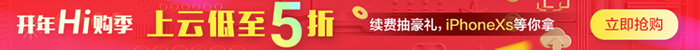#PLSQL 之类型、变量和结构

1. 云栖社区>
2. 博客>
3. 正文## 1、类型

#### 1.1、属性类型

%TYPE：该属性允许在声明中引用数据库中的列或先前声明的变量的数据类型，而不是硬编码类型名称。在声明常量、变量和参数时，都可以使用`%TYPE`属性作为数据类型说明符。如果引用的类型被更改，则声明也将自动更新，这点有利于后期代码维护。

```DECLARE
v_staff_name t_staff.staff_name%TYPE;BEGIN
SELECT t.staff_name INTO v_staff_name FROM demo.t_staff t WHERE t.staff_id=5;
DBMS_OUTPUT.PUT_LINE(v_staff_name);END;```

%ROWTYPE：该属性可以表示数据库中表或游标的行的记录类型。使用`%ROWTYPE`声明的变量可以存储从表中选择或从游标或游标变量获取的整行数据，且变量记录中的字段和每行中的相应列具有相同的名称和数据类型。

```DECLARE
v_staff t_staff%ROWTYPE;BEGIN
SELECT t.* INTO v_staff FROM demo.t_staff t WHERE t.staff_id=5;
DBMS_OUTPUT.PUT_LINE(v_staff.staff_name);END;```

#### 1.2、记录类型

```DECLARE
TYPE staff_type IS RECORD(
staff_name VARCHAR2(50),
gender VARCHAR2(2)
);
v_staff staff_type;BEGIN
SELECT t.staff_name,DECODE(t.gender,1,'男',0,'女','两性') INTO v_staff.staff_name,v_staff.gender  FROM demo.t_staff t WHERE t.staff_id=5;
DBMS_OUTPUT.PUT_LINE(v_staff.staff_name||'|'||v_staff.gender);END;```

## 2、变量

#### 2.2、变量定义

`variable_name datatype [[NOT NULL] {:= | DEFAULT} expression];`

• 1、变量名必须以字母开头。

• 2、变量名长度不能超过 30 个字符。

• 3、变量名中不能含有空格。

• 4、同一语句块内变量名不能重复。

• 5、变量名不能与查询中的列名相同。

#### 2.3、变量赋值

```DECLARE
v1 NUMBER;
v2 NUMBER(5,2);
v3 NUMBER := 50.20;
v4 NUMBER(4) := 1998;
v5 VARCHAR2(4) DEFAULT 'A';
v6 DATE NOT NULL := fn_now;BEGIN
v1 := 100;
v2 := 99.99;
v5 := 'A5';
v6 := SYSDATE;  DBMS_OUTPUT.PUT_LINE(v1||'|'||v2||'|'||v3||'|'||v4||'|'||v5||'|'||v6);END;```

```VARIABLE v1 NUMBERBEGIN
:v1 := 12;
DBMS_OUTPUT.PUT_LINE(:v1);END;/```

## 3、结构

#### 3.2、选择结构

PL/SQL 中提供了两种选择结构，分别是`IF`结构和`CASE`结构。其中`IF`结构有三种变体，`CASE`结构有两种变体。下文将逐一讲述各个选择语句：

IF 结构变体一：

```IF condition THEN
{...statements to execute when condition is TRUE...}END IF;```

```BEGIN
IF 1>0 THEN
DBMS_OUTPUT.PUT_LINE('executed');  END IF;END;```

IF 结构变体二：

```IF condition THEN
{...statements to execute when condition is TRUE...}ELSE
{...statements to execute when condition is FALSE...}END IF;```

```BEGIN
IF 1>2 THEN
DBMS_OUTPUT.PUT_LINE('The result is true');
ELSE
DBMS_OUTPUT.PUT_LINE('The result is false');  END IF;END;```

IF 结构变体三：

```IF condition1 THEN
{...statements to execute when condition1 is TRUE...}ELSIF condition2 THEN
{...statements to execute when condition2 is TRUE...}[ELSE
{...statements to execute when both condition1 and condition2 are FALSE...}]END IF;```

```BEGIN
IF 1>2 THEN
DBMS_OUTPUT.PUT_LINE('1>2 branch');
ELSIF 1<2 THEN
DBMS_OUTPUT.PUT_LINE('1<2 branch');  ELSE
DBMS_OUTPUT.PUT_LINE('1=2 branch');  END IF;END;```

CASE 结构变体一

```DECLARE
WHEN 'A' THEN DBMS_OUTPUT.PUT_LINE('甲');
WHEN 'B' THEN DBMS_OUTPUT.PUT_LINE('乙');
WHEN 'C' THEN DBMS_OUTPUT.PUT_LINE('丙');    ELSE DBMS_OUTPUT.PUT_LINE('丁');  END CASE;END;```

CASE 结构变体二

```DECLARE
v_score NUMBER(3);
BEGIN
v_score:=78;  CASE
WHEN v_score>=80 THEN DBMS_OUTPUT.PUT_LINE('优');
WHEN v_score>=70 THEN DBMS_OUTPUT.PUT_LINE('良');
WHEN v_score>=60 THEN DBMS_OUTPUT.PUT_LINE('中');
ELSE DBMS_OUTPUT.PUT_LINE('差');
END CASE;END;```

`ELSE RAISE CASE_NOT_FOUND;`

#### 3.3、循环结构

PL/SQL 中提供了三种循环结构，分别是`LOOP``WHILE LOOP``FOR LOOP`。另外 PL/SQL 还提供了`EXIT`语句用于退出当前循环。下文将逐一讲述各个循环语句：

LOOP 循环

```LOOP  {...statements...}
EXIT [ WHEN boolean_condition ];END LOOP;```

```DECLARE
v_counter BINARY_INTEGER := 0;
BEGIN  LOOP
v_counter := v_counter + 1;
DBMS_OUTPUT.PUT_LINE(v_counter); -- 输出结果：1、2、3、4、5、6、7、8、9
IF v_counter >= 9 THEN
EXIT;    END IF;
-- 上面的 IF 语句块还可以由“EXIT WHEN v_counter >= 9;”代替  END LOOP;END;```

```DECLARE  i BINARY_INTEGER := 0;  j BINARY_INTEGER := 0;
BEGIN
LOOP    i := i + 1;    j := 0;

LOOP      j := j + 1;
DBMS_OUTPUT.PUT_LINE('i*j=('||i||'*'||j||')='||i*j);
EXIT WHEN j >= 3;
END LOOP;

EXIT WHEN i >= 4;
END LOOP;
END;```

```DECLARE  i BINARY_INTEGER := 0;  j BINARY_INTEGER := 0;
BEGIN
<<outer_loop>>
LOOP    i := i + 1;    j := 0;
<<inner_loop>>
LOOP      j := j + 1;
DBMS_OUTPUT.PUT_LINE('i*j=('||i||'*'||j||')='||i*j);
EXIT inner_loop WHEN j >= 3;
EXIT outer_loop WHEN i >= 4;
END LOOP inner_loop;
END LOOP outer_loop;
END;```

WHILE LOOP 循环

```WHILE condition
LOOP  {...statements...}END LOOP;```

```DECLARE
v_score NUMBER(3) := 0;BEGIN
WHILE v_score < 60 LOOP
v_score := v_score + 10;
DBMS_OUTPUT.PUT_LINE(v_score); -- 输出结果：10、20、30、40、50、60
END LOOP;
DBMS_OUTPUT.PUT_LINE('over');END;```

FOR LOOP 循环

```FOR loop_counter IN [REVERSE] lowest_number..highest_number
LOOP  {...statements...}END LOOP;```

```BEGIN
FOR i IN 3..7 LOOP
DBMS_OUTPUT.PUT_LINE(i); -- 输出结果：3、4、5、6、7
END LOOP;END;```

```BEGIN
FOR i IN REVERSE 3..7 LOOP
DBMS_OUTPUT.PUT_LINE(i); -- 输出结果：7、6、5、4、3
END LOOP;END;```

【云栖快讯】一站式开发者服务，海量学习资源免费学  详情请点击

+ 关注